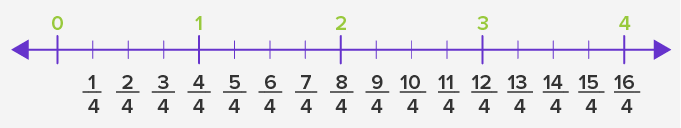# Fraction Number Line - Definition with Examples

The Complete K-5 Math Learning Program Built for Your Child

• 40 Million Kids

Loved by kids and parent worldwide

• 50,000 Schools

Trusted by teachers across schools

• Comprehensive Curriculum

Aligned to Common Core

## What is Fraction Number Line?

Fraction: A fraction denotes a part of a whole or a collection.

For example,

There are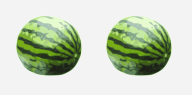. I give 1 of them to my friend.

I’m left with.

I eatof it. What am I left with?

Of course, the remainingThe remaining watermelon is nothing but a fraction. It is called half.

Half is denoted as 1, where 1 is the numerator and 2 is the denominator.

Number Line: In math, a number line is a straight line with numbers placed at equal intervals or segments along its length.

A number line can be extended infinitely in any direction, and we usually represent it horizontally. The numbers on the number line increase as we move from left to right and decrease moving from right to left.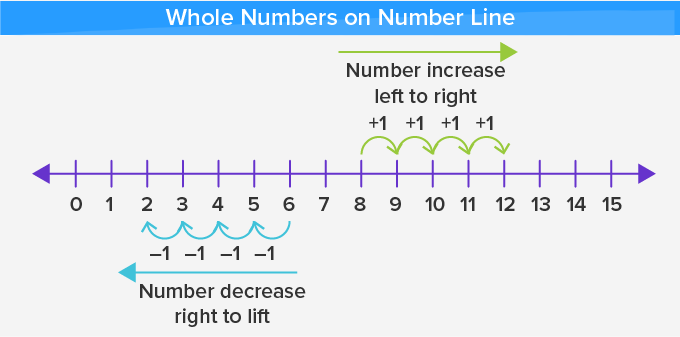## Fraction Number Line

We know a fraction is less than 1.

So, if we have watermelon cut in half as shown below: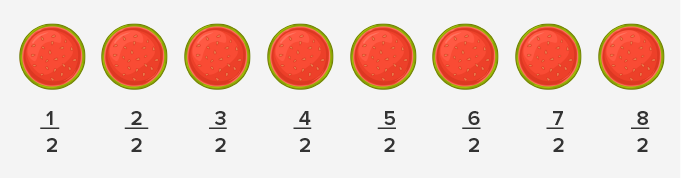We can represent them on a number line by following the given steps.

1. We draw a number line with markings at equal intervals.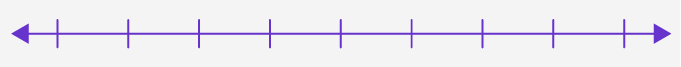2. Then label the marks as shown.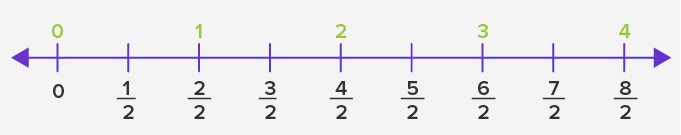## Steps to make a fraction number line:

Step 1: Draw a number line with whole numbers.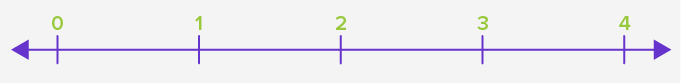Step 2: Look for the denominator of the fractions we want to show on the number line.

For example, to show fourths on the number line.

Step 3: Mark as many points between each whole division as the denominator.

For example, to show fourths, divide each unit division into 4 parts.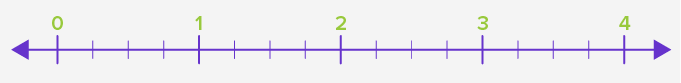Step 4: Write the fractions with the denominator.

For example, we want to show fourths, So, the denominator of the fractions will be 4.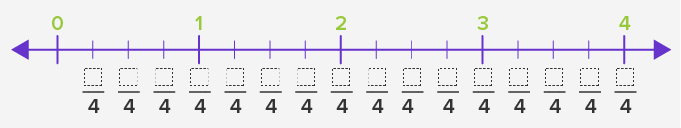Step 5: Write the numerators as 1, 2, 3, 4, and so on.